# [论文速览] RectifiedFlow@Flow Straight and Fast{colon}Learning to Generate and Transfer Data with Rectified Flow

## Pre

title: Flow Straight and Fast: Learning to Generate and Transfer Data with Rectified Flow
accepted: ICLR 2023
paper: https://arxiv.org/abs/2209.03003 / https://openreview.net/forum?id=XVjTT1nw5z
code: https://github.com/gnobitab/RectifiedFlow
ref: https://zhuanlan.zhihu.com/p/603740431
ref: https://www.spaces.ac.cn/archives/9497

ps. 速度含方向，是矢量

## Motivation&Solution

1. 各类无监督学习共同的困难是缺少配对的输入输出数据，它们大多数都希望寻找两个分布的数据点之间的关系
2. Diffusion Generative Models(扩散式生成模型)效果很好，但生成速度缓慢，数学推导复杂，依赖随机微分方程(Stochastic Differential Equation (SDE))的知识。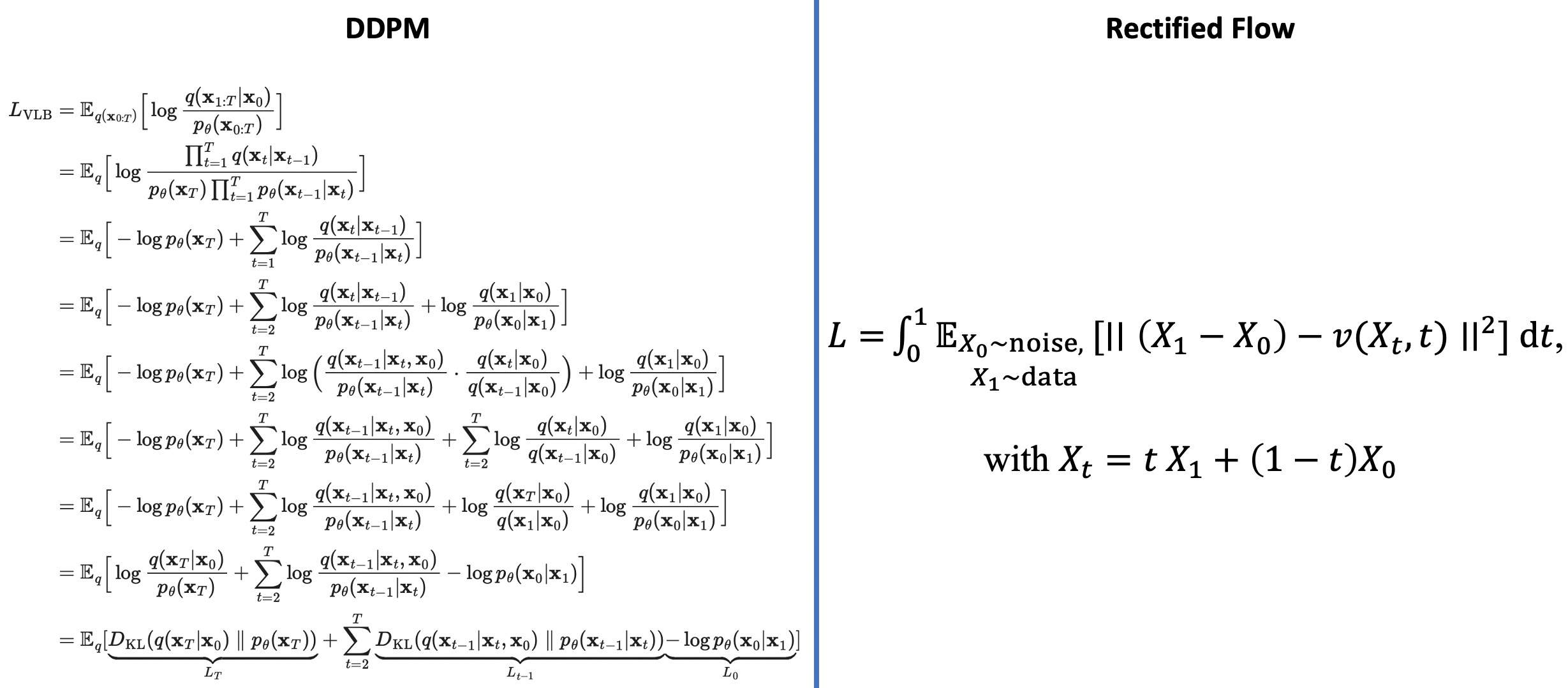1. Motivation1: 通常的扩散模型把高斯白噪声转换成想要的数据(比如图片)。本方法可以把任何一种数据(比如猫脸照片)或噪声转换成另外一种数据(比如人脸照片)。不仅可以做生成模型，还可以应用于很多更广泛的迁移学习 (比如domain transfer）任务上。
2. Motivation2: 基于简单的常微分方程ODE(Ordinary Differential Equation)，不需要变分法或随机微分方程SDE(Stochastic Differential Equation)的知识，概念简单，推理时更快速
3. Motivation2: 使用reflow方法实现“一步生成，快速，计算量小（对比扩散模型）

## Background

The Transport Mapping Problem 给定两个分布，找一个传输映射T可以将 $$x_0$$ 映射到另一个分布的数据点 $$x_1$$。在传统的生成模型中，T参数化为一个神经网络，用GAN类的最小最大化算法或最大似然估计（MLE）进行训练。

$\max_v \mathbb{D}(\rho^{v,\pi_{0}}) \tag{19}$

models 和 DDPM，统称降噪扩散方法，他们没有GAN的那些问题，而且学好的SDEs还能通过 probability flow ODEs 和 DDIM 的方法转换成确定性的ODE模型，以实现更快的推理。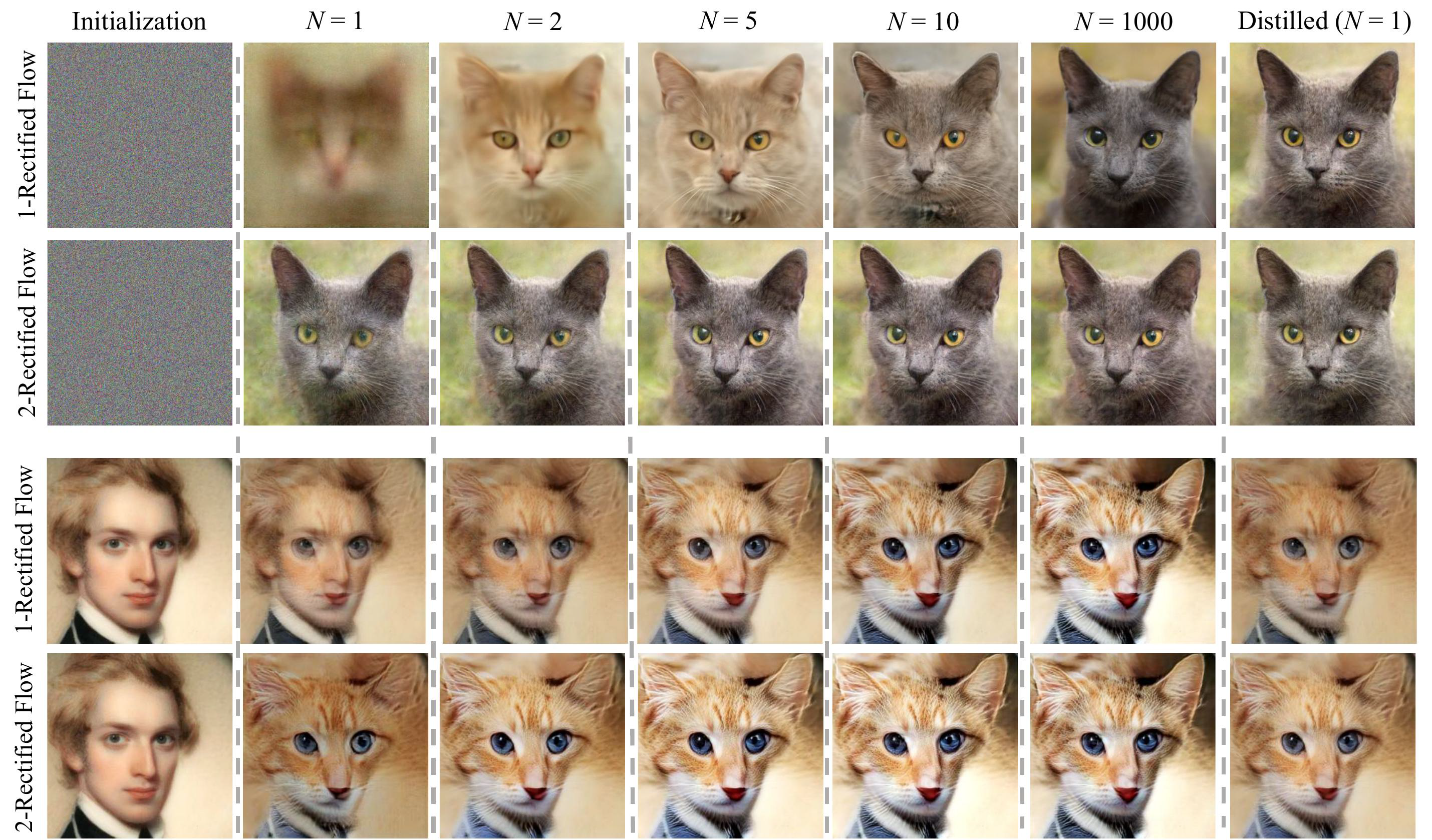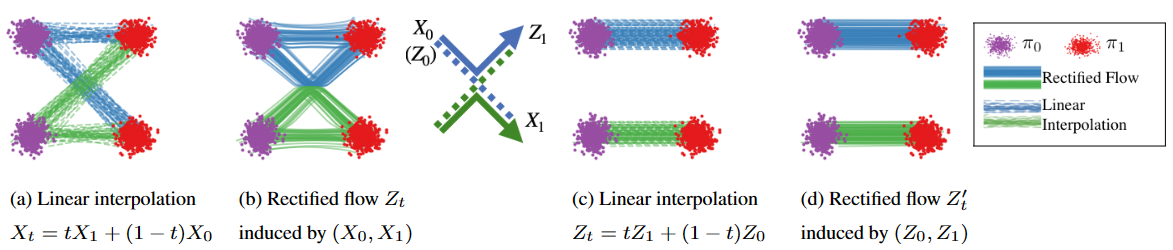Unpaired Image-to-Image translation standard denoising diffusion、PF-ODEs关注生成任务，要做I2I的话可以用两个PF-ODEs将source转换为latent，再把latent换成target，或者用什么energy-guided方法。而rectified flow单纯地把 $$\pi_0$$ 的噪声换成source domain就好了

Re-thinking the role of diffusion noise 本文工作展示了如果希望学习ODEs，那么无需利用SDE工具，并比较了二者的差别：

1. ODE概念简单且计算更快
2. ODE前向反向计算一样容易，SDE很难沿时间反向
3. ODE学到的$$Z_0,Z_1$$更确定且传输代价可以用rectified flow降低，提供更好的隐空间做表征或操控输出
4. ODE训练不会比SDE更难
5. 二者表达边缘分布的能力一样强，但需要更丰富的时间相关结构时，更偏好SDEs
6. 流形数据ODE的输出往往落入平滑的低维流形，这是人工智能中结构化数据（如图像和文本）的关键归纳。而SDE会得到平滑的结果，导致计算缓慢，而且有调整超参的负担，它更适合建模高噪声数据，如金融经济领域，或分子模拟那样涉及物理上的扩散过程的领域。

## Method（Model）

### Overview

$\begin{equation}\frac{d\boldsymbol{x}_t}{dt}=\boldsymbol{f}_t(\boldsymbol{x}_t) \end{equation}$

${\frac{d}{d t}}Z_{t}=v(Z_{t},t),~~Z_{0}\sim\pi_{0},~\forall t\in[0,1].$

$Z_{t+\epsilon}=Z_{t}+\epsilon v(Z_{t},t),$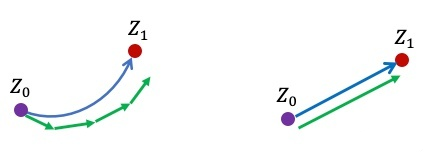$x_{t}=t x_{1}+(1-t)x_{0}, t\in[0,1].$

${\frac{d}{d t}}x_{t} = x_{1} - x_{0}$

$\min_{v} \int_{0}^{1}\mathbb{E}_{x_0\sim\pi_0,x_1\sim\pi_1}\left[\left|\left|(x_{1}-x_{0})-v(x_{t},t)\right|\right|^{2}\right]d t,\quad with\quad x_{t}=t x_{1} + (1-t)x_0$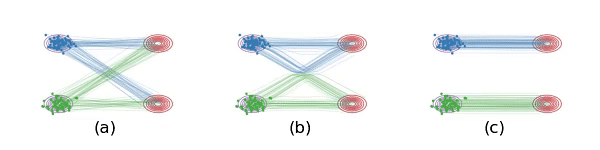$\min_{v} \int_{0}^{1}\mathbb{E}_{x_0\sim\pi_0,x_1\sim Flow_1(x_0)}\left[\left|\left|(x_{1}-x_{0})-v(x_{t},t)\right|\right|^{2}\right]d t,\quad where\quad x_{t}=t x_{1} + (1-t)x_0$

2-Rectified Flow和1-Rectified Flow在训练过程中唯一的区别就是数据配对不同，上图的c就展示了Reflow的效果，此时 $$(x_0,\; x_1)$$ 更配对，直线插值交叉数减少，但实际上仔细看还不是特别直。理论上可以重复Reflow多次，可证明该过程其实是在单调地减小最优传输理论中的传输代价(transport cost)，而且最终收敛到完全直的状态。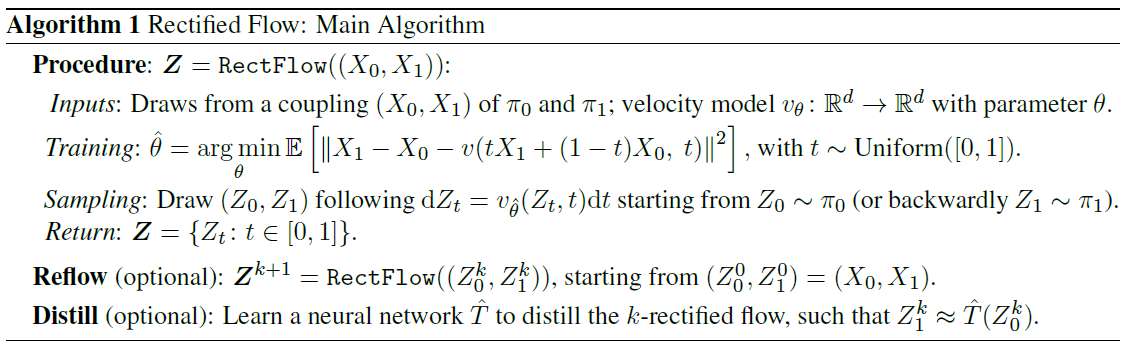### Main Results and Properties

Reducing transport costs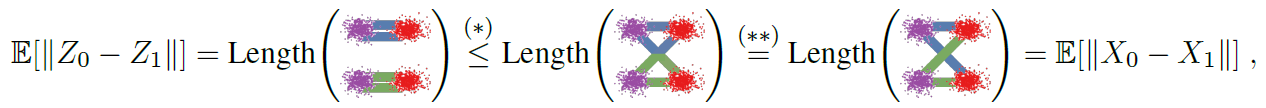Reflow, straightening, fast simulation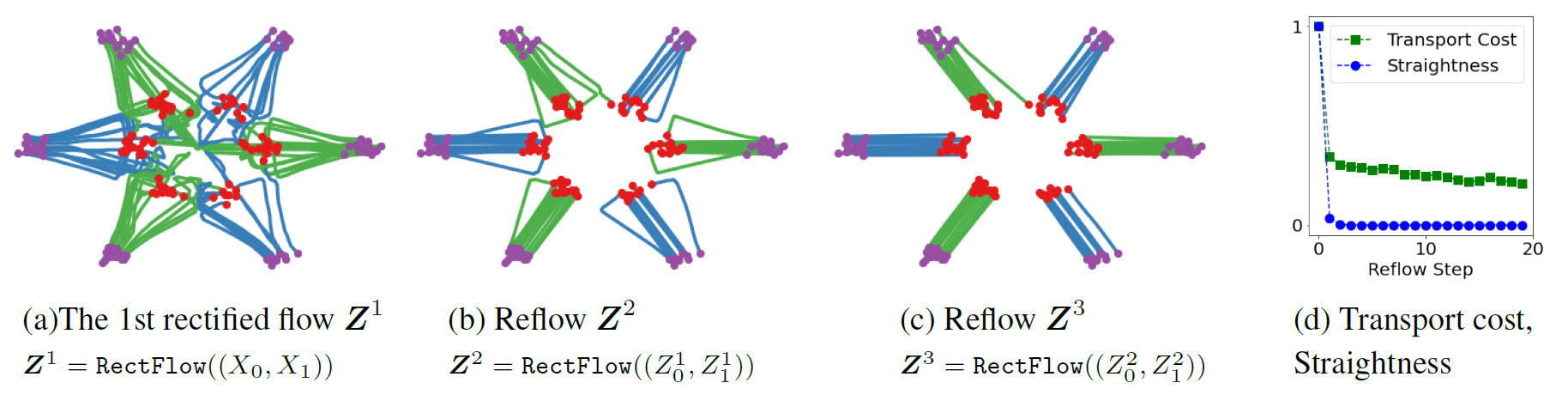$S(Z) = \int_{0}^{1}\mathbb{E}\left[\left|\left|(Z_{1}-Z_{0})-\dot{Z}_t\right|\right|^{2}\right]dt. \tag{3}$

$$S(Z) = 0$$ 时为直，但还是想知道为什么会不直

Distillation
distillation会忠实地逼近 $$(Z^k_o,Z^k_1)$$ 对，而rectification则会产生更直的流以及更低传输代价的 $$(Z^{k+1}_o,Z^{k+1}_1)$$ ，因此distillation只能用于最终阶段

Probability Flow ODEs and DDIM 概率流模型：probability flow ODEs (PF-ODEs)，作者说所有PF-ODEs的变种都可以看成是 $$X_{t}=\alpha_{t}X_{1}+\beta_{t}\xi$$ 的形式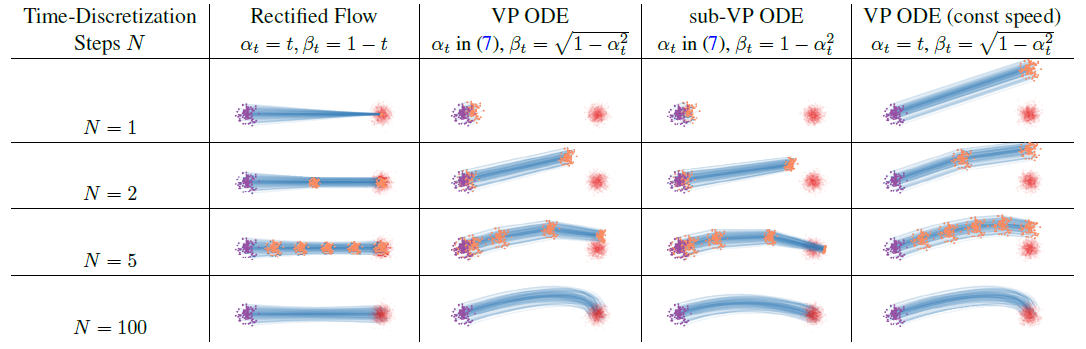### Theoretical Analysis

The Straightening Effect 只有线性插值路径不相交时才说配对是直的（coupling is straight）
Straight vs. Optimal Couplings 拉直是必要的，但不是最优配对的充分条件
Denoising Diffusion Models and Probability Flow ODEs PF-ODEs可以看做是非线性的 rectified flows

### 一些补充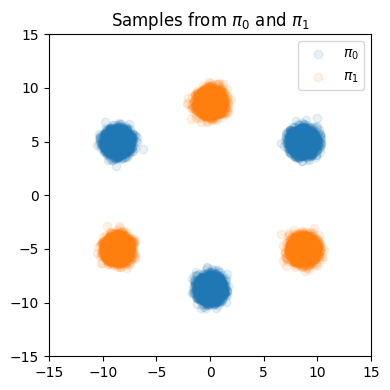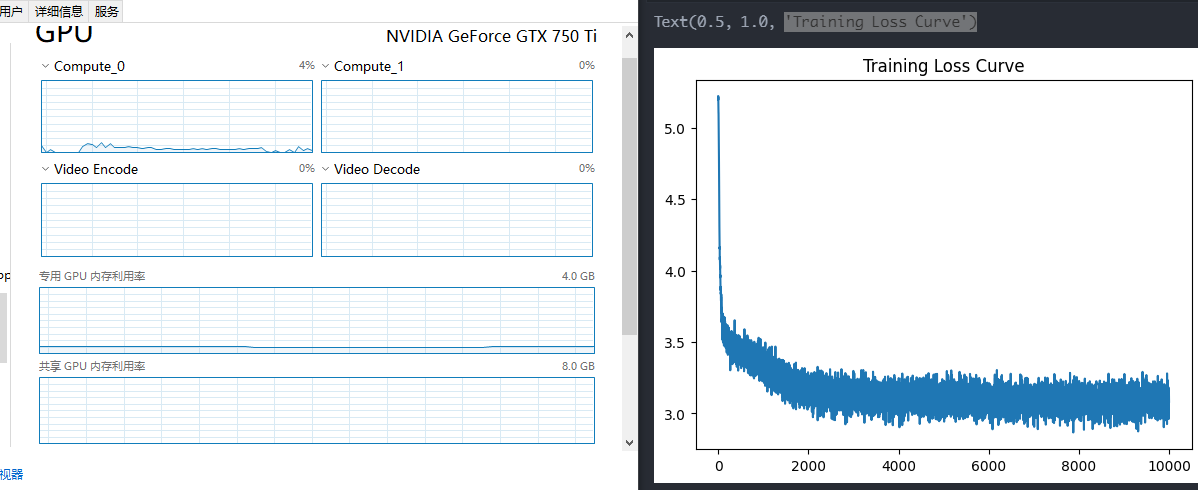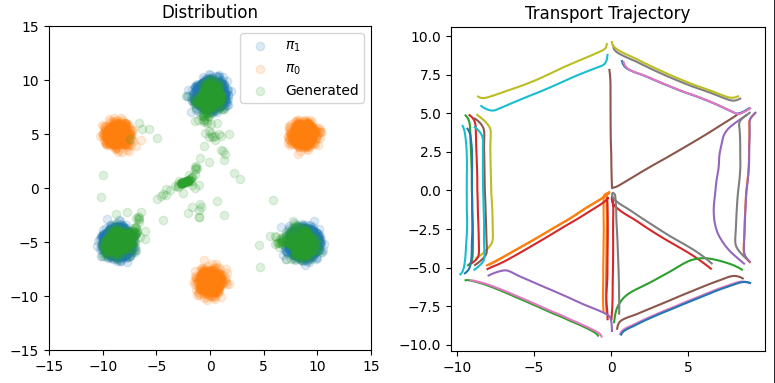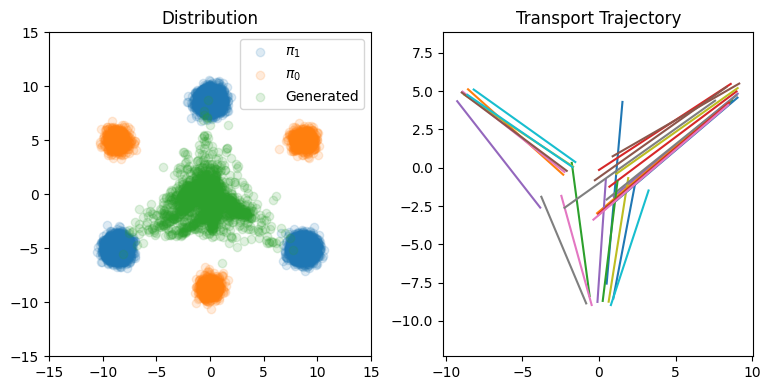$Z_{t+\epsilon}=Z_{t}+\epsilon v(Z_{t},t),$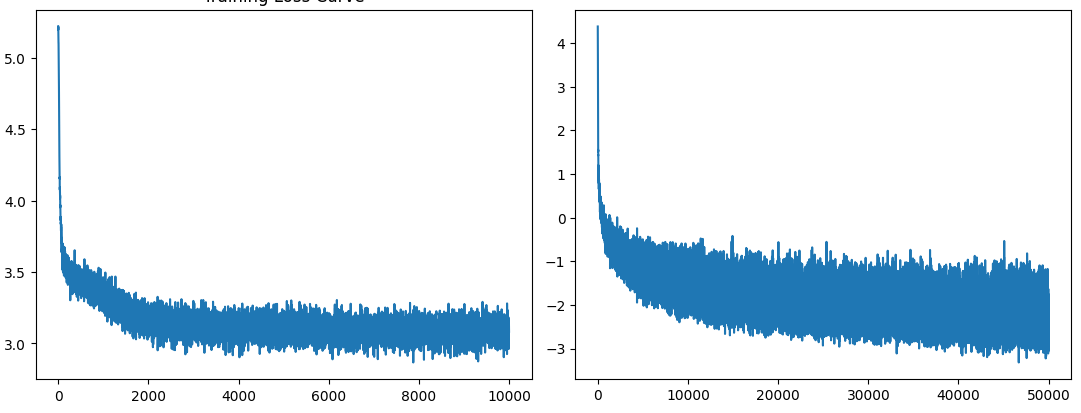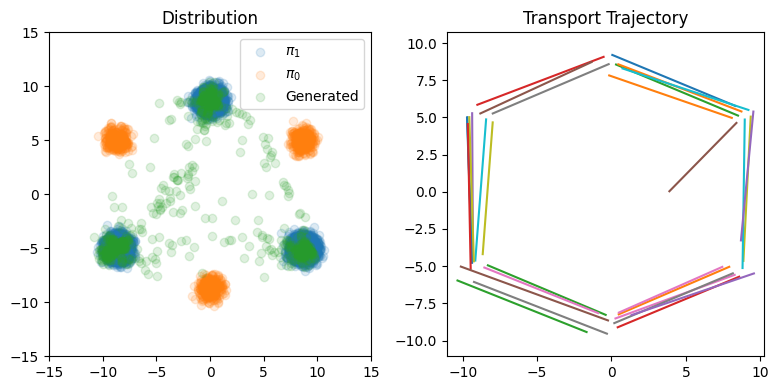## Experiment

### Training Detail

Image-to-Image Translation 在不提供配对样本的情况下转换风格，并保持主体目标的identity

$$h(x)$$表示风格图片x的特征映射，$$X_{t}=tX_{1}+(1-t)X_{0}$$，有$$H_t = h(X_t),\; \mathrm{d}H_{t} = \nabla h(X_{t})^{T}(X_{1} - X_{0})\mathrm{d}t$$。要想风格转换正确，可以通过学习到的模型得到$$H'_t = h(Z_t)$$，它尽可能地逼近$$H_t$$。因为$$\mathrm{d}H_{t}^{\prime} = \nabla h(Z_{t})^{T}v(Z_{t},t)\mathrm{d}t$$，可最小化下列损失：

$\min_{v}\int_{0}^{1}\mathbb{E}\left[\left|\left|\nabla h(X_{t})^{\top}(X_{1}-X_{0}-v(X_{t},t))\right|\right|_{2}^{2}\right]\mathrm{d}t, \qquad X_{t}=t X_{1}+(1-t)X_{0}. \tag{20}$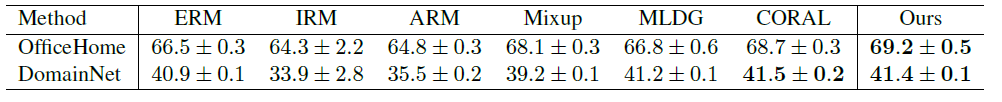### Dataset

Image Generation, I2I: CIFAR-10 和高分辨率数据集 LSUN Bedroom, LSUN Church, CelebA HQ, AFHQ Cat

### Results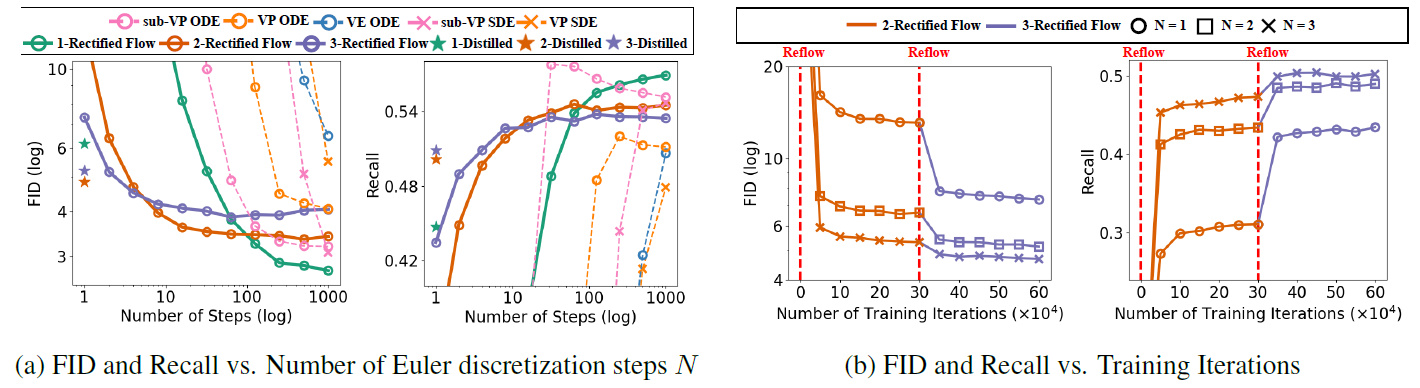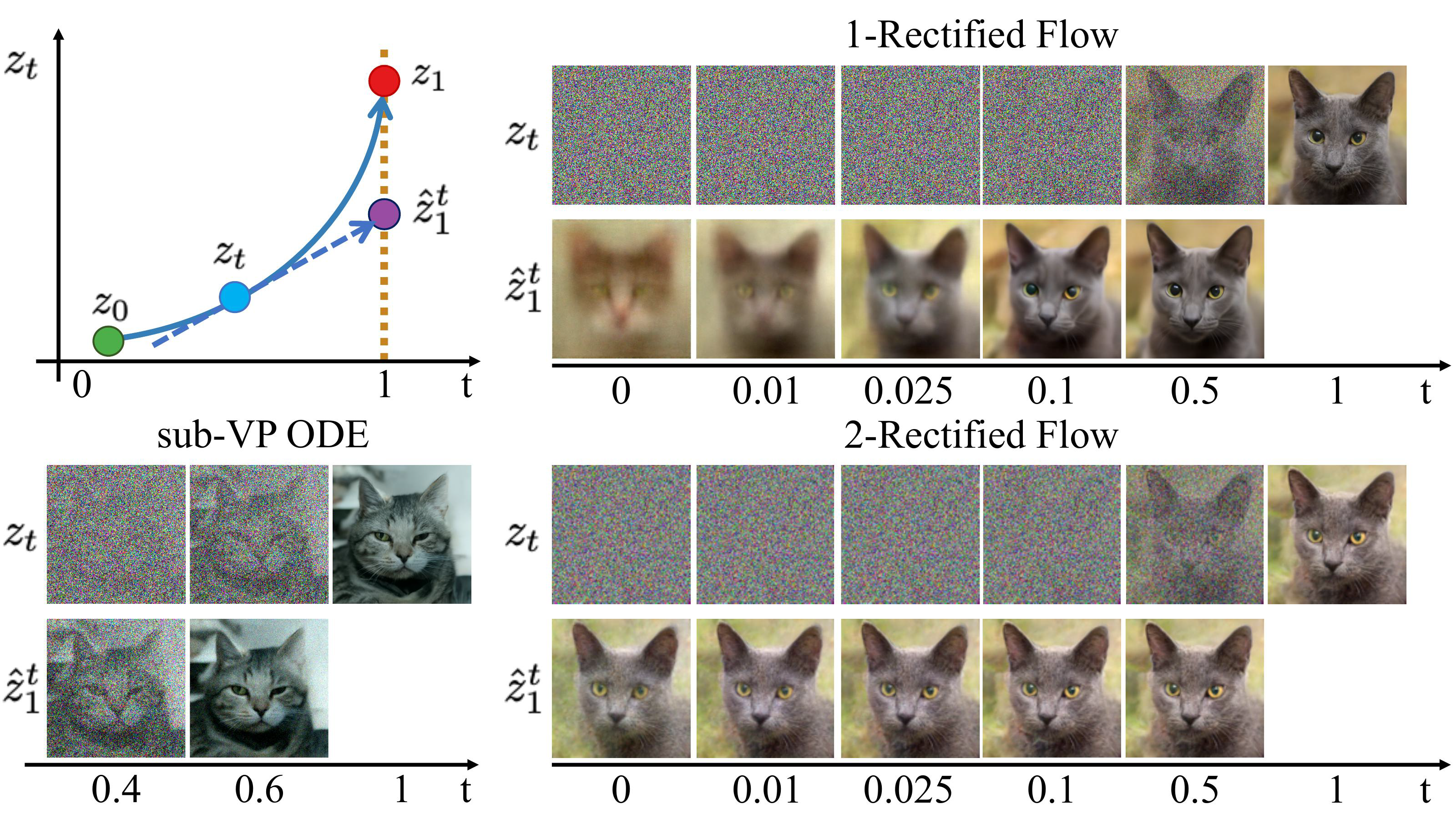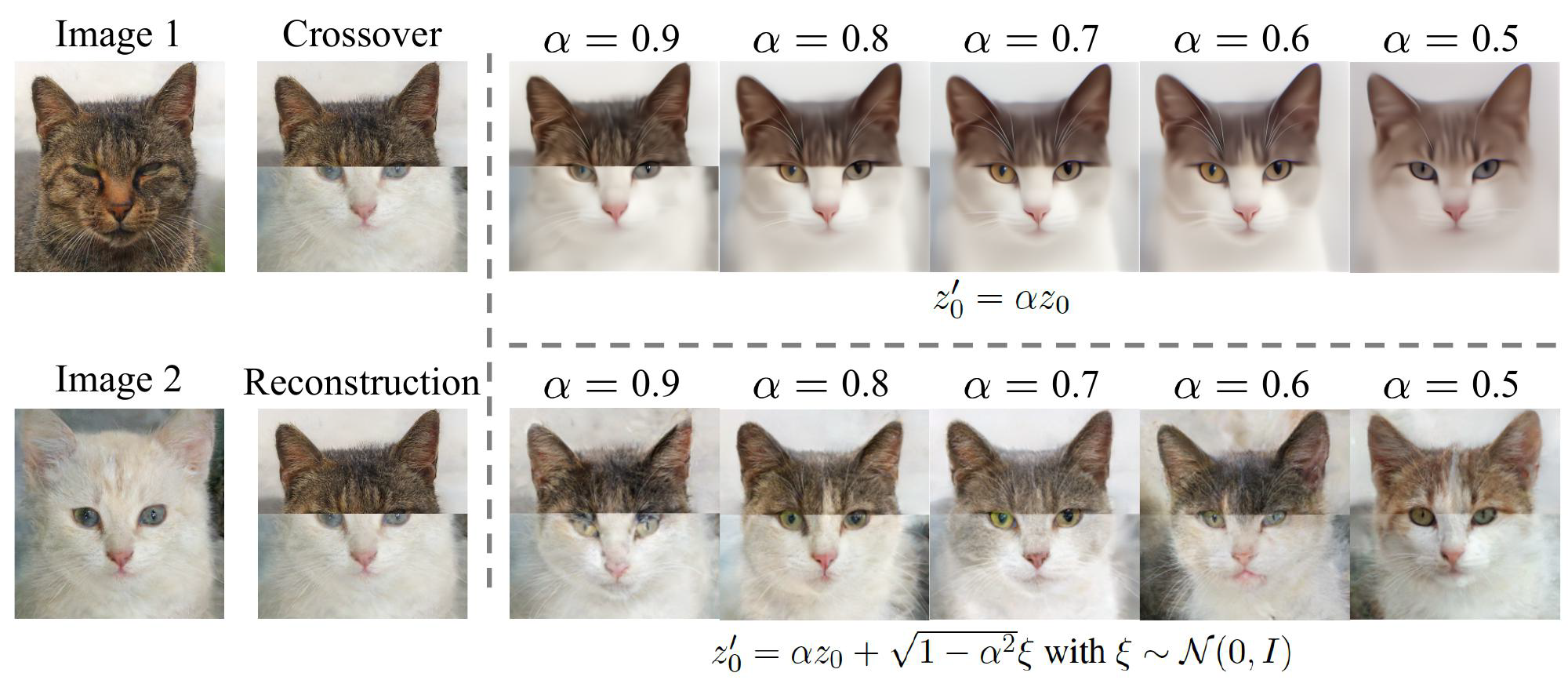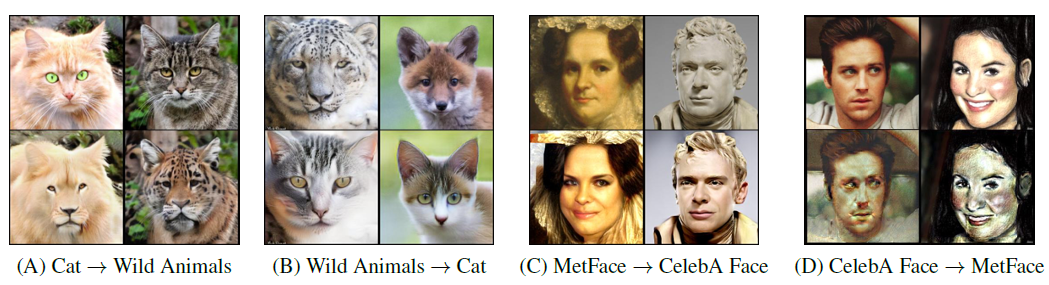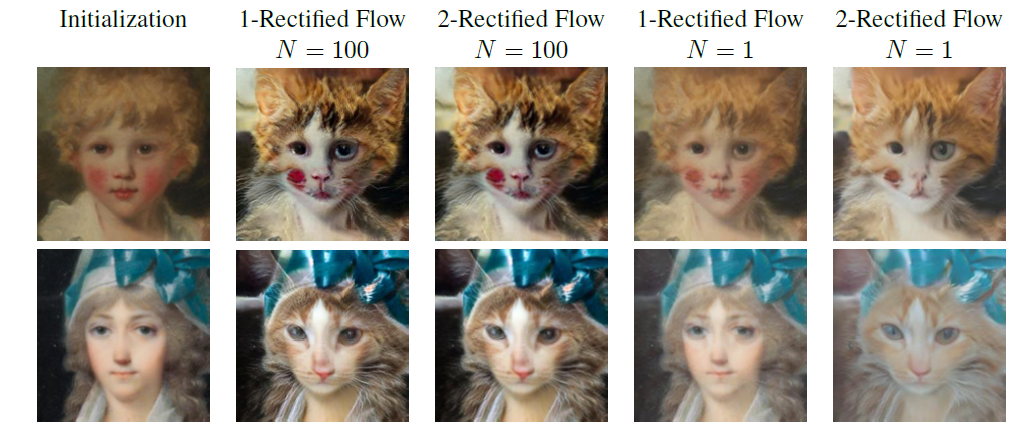## Unknown

• 如何理解”非因果 = 轨迹交叉 = ODE解不唯一“？
• 图t1 作者1-rectified flow是(a), 还是(b)
posted @ 2023-05-26 14:56  NoNoe  阅读(111)  评论(0编辑  收藏  举报